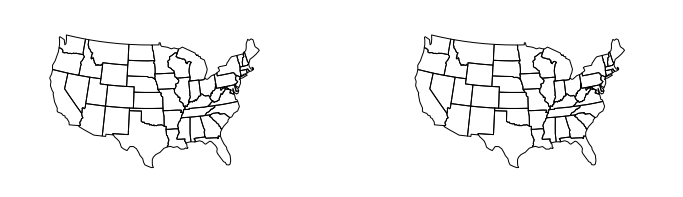# 使用投影¶

## 投影与非投影¶

```import geopandas as gpd
import geoplot as gplt
%matplotlib inline

gplt.polyplot(contiguous_usa)
```
```<matplotlib.axes._subplots.AxesSubplot at 0x11a914208>
```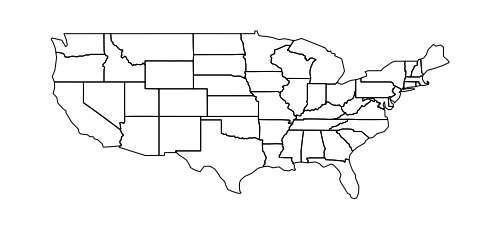```boroughs = gpd.read_file(gplt.datasets.get_path('nyc_boroughs'))
gplt.polyplot(boroughs)
```
```<matplotlib.axes._subplots.AxesSubplot at 0x11d243898>
```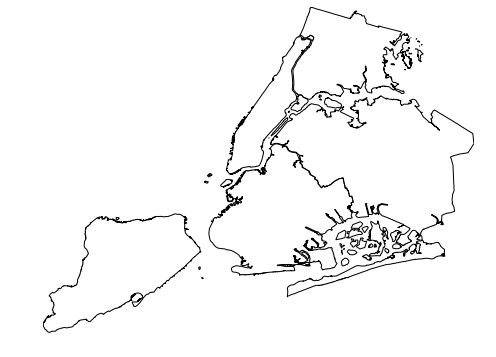```import geoplot.crs as gcrs
gplt.polyplot(contiguous_usa, projection=gcrs.AlbersEqualArea())
```
```<cartopy.mpl.geoaxes.GeoAxesSubplot at 0x11dd02cc0>
```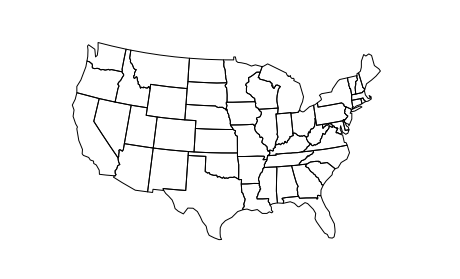## 叠加投影图¶

```cities = gpd.read_file(gplt.datasets.get_path('usa_cities'))
ax = gplt.polyplot(
contiguous_usa,
projection=gcrs.AlbersEqualArea()
)
gplt.pointplot(cities, ax=ax)
```
```<cartopy.mpl.geoaxes.GeoAxesSubplot at 0x11da21c50>
```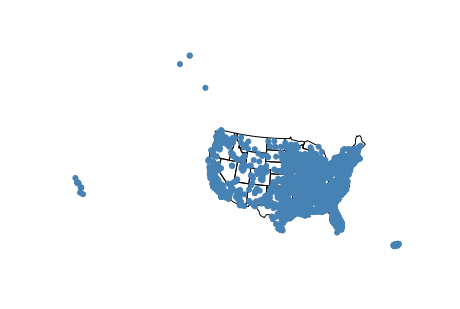```ax = gplt.polyplot(
contiguous_usa,
projection=gcrs.AlbersEqualArea()
)
gplt.pointplot(cities, ax=ax, extent=contiguous_usa.total_bounds)
```
```<cartopy.mpl.geoaxes.GeoAxesSubplot at 0x11da947f0>
```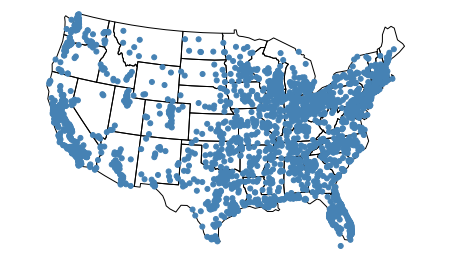## 子地块上的投影¶

```import matplotlib.pyplot as plt
import geoplot as gplt

f, axarr = plt.subplots(1, 2, figsize=(12, 4))
gplt.polyplot(contiguous_usa, ax=axarr)
gplt.polyplot(contiguous_usa, ax=axarr)
```
```<matplotlib.axes._subplots.AxesSubplot at 0x11dc55438>
```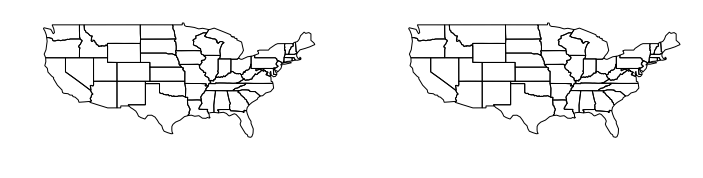`matplotlib` 支持使用 `projection` 参数 `subplot_kw` .

```proj = gcrs.AlbersEqualArea(central_longitude=-98, central_latitude=39.5)
f, axarr = plt.subplots(1, 2, figsize=(12, 4), subplot_kw={
'projection': proj
})
gplt.polyplot(contiguous_usa, projection=proj, ax=axarr)
gplt.polyplot(contiguous_usa, projection=proj, ax=axarr)
```
```<cartopy.mpl.geoaxes.GeoAxesSubplot at 0x11ded2b70>
```